Examples

Chapter 13 Class 9 Surface Areas and Volumes
Serial order wise

For calculations, Lateral Surface Area means curved surface area. It's called lateral surface area for cube and cuboid and curved surface area for cylinder, cone and hemisphere.

The formulas for calculating Surface Area and Volume are

## Cube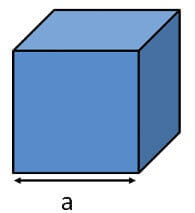• Lateral Surface Area = 4a 2
• Total Surface Area = 6a 2
• Volume = a 3

## Cuboid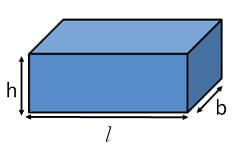• Lateral Surface Area = 2(lh + bh) = 2 (l + b) h
• Total Surface Area = 2(lb + bh + lh)
• Volume = l x b x h

## Cylinder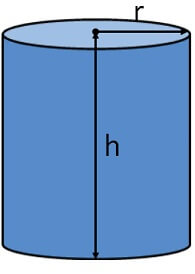• Curved Surface Area = 2πrh
• Total Surface Area = 2πrh + πr 2  + πr 2 = 2πrh + 2πr 2  = 2πr(r + h)
• Volume = πr 2 h

## Cone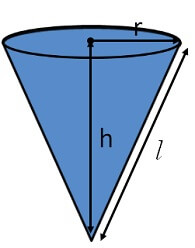• Curved Surface Area = πrl
• Total Surface Area = πrl + πr 2  = πr(r + l)
• Volume = 1/3 πr 2 h

## Hemisphere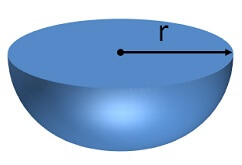• Curved Surface Area = 2πr 2
• Total Surface Area = 2πr 2 + πr 2  = 3πr 2
• Volume = 2/3 πr 3

## Sphere• Surface Area = 4πr 2
• Volume = 4/3 πr 3

## Frustum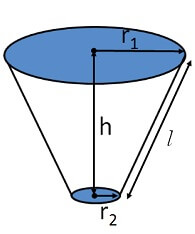• Curved Surface Area = π(r 1 + r 2 )l
• Total Surface Area =π(r 1 + r 2 )l + πr 1 + πr 2 2
• Volume = 1/3 πh (r 1 2 + r 2 2 + r 1 r 2 )

Maths Crash Course - Live lectures + all videos + Real time Doubt solving!